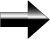Integration

Integration can be considered the opposite of differentiation and is hence the other of the two main principles of calculus.

When we integrate a function, we are finding the area between the function and the x-axis.  We must also specify the limits of integration, which tells us the boundaries of the area in terms of the horizontal direction.

We estimate the area under the curve by using rectangles.  The total area under the curve is approximately the total area of all the rectangles.  We can see that in this case it is an underestimation.

We can see that the more rectangles we use, the better our estimation of the area.  If we take the limit as either the number of rectangles, n, goes to infinity, or if Δx goes to zero, then our estimation of the area will be exact

This is the concept of integration.

Main Calculus Page